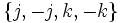# Power automorphism not implies universal power automorphism

This article gives the statement and possibly, proof, of a non-implication relation between two automorphism properties. That is, it states that every automorphism satisfying the first automorphism property (i.e., power automorphism) need not satisfy the second automorphism property (i.e., universal power automorphism)
View a complete list of automorphism property non-implications | View a complete list of automorphism property implications
Get more facts about power automorphism|Get more facts about universal power automorphism

## Statement

A power automorphism of a group (i.e., an automorphism that sends every element to a power of itself) need not be a universal power automorphism.

## Definitions used

### Power automorphism

Further information: Power automorphism, power map

Let$G$ be a group. An automorphism$\sigma$ of$G$ is termed a power automorphism of$G$ if, for every$g \in G$, there exists a$n$ such that$\sigma(g) = g^n$.

### Universal power automorphism

Further information: Universal power automorphism, universal power map

Let$G$ be a group. An automorphism$\sigma$ of$G$ is termed a universal power automorphism (or a uniform power automorphism) if there exists a$n$ such that$\sigma(g) = g^n$ for all$g \in G$.

## Proof

### Example of the quaternion group

In the quaternion group:$\{ 1,-1,i,-i,j,-j,k,-k \}$

conjugation by$i$ is a power automorphism that is not a universal power automorphism.

• It is a power automorphism: It fixes$\{1,-1,i,-i \}$, and sends the other elements to their inverses.
• It is not a universal power automorphism: It sends the elements$\{j,-j,k,-k\}$ to their inverses. Since each of these elements have order four, any$n$ for which this automorphism is the$n^{th}$ power map, must be congruent to$-1$ mod$4$. On the other hand, since it fixes$i$, which also has order four,$n$ must be$1$ modulo$4$, a contradiction.